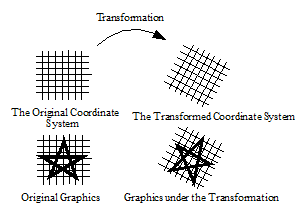### 3.4 Transformations in CLIM

One of the features of CLIM's graphical capabilities is the use of coordinate system transformations. By using transformations, you can often write simpler graphics code because you can choose a coordinate system in which to express the graphics that simplifies the description of the drawing.

A transformation is an object that describes how one coordinate system is related to another. A graphic function performs its drawing in the current coordinate system of the stream. A new coordinate system is defined by describing its relationship to the old one (the transformation). The drawing can now take place in the new coordinate system. The basic concept of graphic transformations is illustrated in Figure 16..###### Figure 16. Graphic Transformation

For example, you might define the coordinates of a five-pointed star and a function to draw it.

`(defvar *star* '(0 3 2 -3 -3 1/2 3 1/2 -2 -3))`
` `
`(defun draw-star (stream) `
`  (clim:draw-polygon* stream *star* :closed t :filled nil)) `

Without any transformation, the function draws a small star centered around the origin. By applying a transformation, the same function can be used to draw a star of any size, anywhere. For example:

`(clim:with-room-for-graphics (stream)`
` (clim:with-translation (stream 100 100) `
`                        (clim:with-scaling (stream 10)`
`                         (draw-star stream))) `
` (clim:with-translation (stream 240 110)`
`                        (clim:with-rotation (stream -0.5)`
`                         (clim:with-scaling (stream 12 8)`
`                          (draw-star stream))))) `

will draw a picture somewhat like Figure 16. on ``` stream``` .

Common Lisp Interface Manager 2.0 User's Guide - 7 Aug 2017